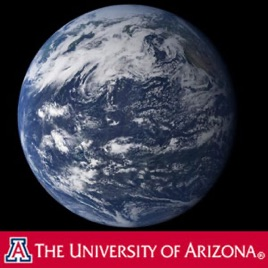59 сек.# Time and Space 7. Geology and Physics

• Естественные науки

Transcript: In the physics of Isaac Newton, time and space are linear and absolute. Einstein’s theories of relativity changed forever our notions of time and space. In Einstein's special theory of relativity the equivalence of mass and energy and the idea that the speed of light is a fundamental constant means that time and space must be supple. Time can slow down due to high speed travel near the velocity of light. Masses can increase, and physical lengths can actually contract. These effects are real and observed in physics labs every day. In the general theory of relativity we have the idea that mass curves space. We can see this because photons must have an equivalent mass, and so photons in the presence of another gravitating object must be affected by that object. Mass curves light.

Transcript: In the physics of Isaac Newton, time and space are linear and absolute. Einstein’s theories of relativity changed forever our notions of time and space. In Einstein's special theory of relativity the equivalence of mass and energy and the idea that the speed of light is a fundamental constant means that time and space must be supple. Time can slow down due to high speed travel near the velocity of light. Masses can increase, and physical lengths can actually contract. These effects are real and observed in physics labs every day. In the general theory of relativity we have the idea that mass curves space. We can see this because photons must have an equivalent mass, and so photons in the presence of another gravitating object must be affected by that object. Mass curves light.

59 сек.